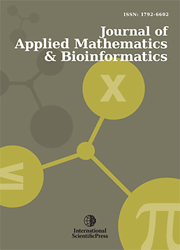# Journal of Applied Mathematics & Bioinformatics

#### Bayesian Investigation of Principal Components Using Mixture Priors

•[ Download ]
• Times downloaded: 1787
• Abstract

Bayesian approach for principal component analysis (PCA) is a novel method to determine the number of dimensionality through using different prior probabilities. In common strategy one often equally selects variances for the columns of mapping matrix by using the mixture priors. In this article, we generalize this approach by using the mixture priors with different variances in multivariate normal distribution. Further, we employ an orthogonal transformation to convert a set of observations of possibly correlated variables into a set of values of linearly uncorrelated variables. Estimations of principal component and number of effective dimensionality are performed via Markov Chain Monte Carlo (MCMC) algorithm.ISSN: 1792-6939 (Online)
1792-6602 (Print)